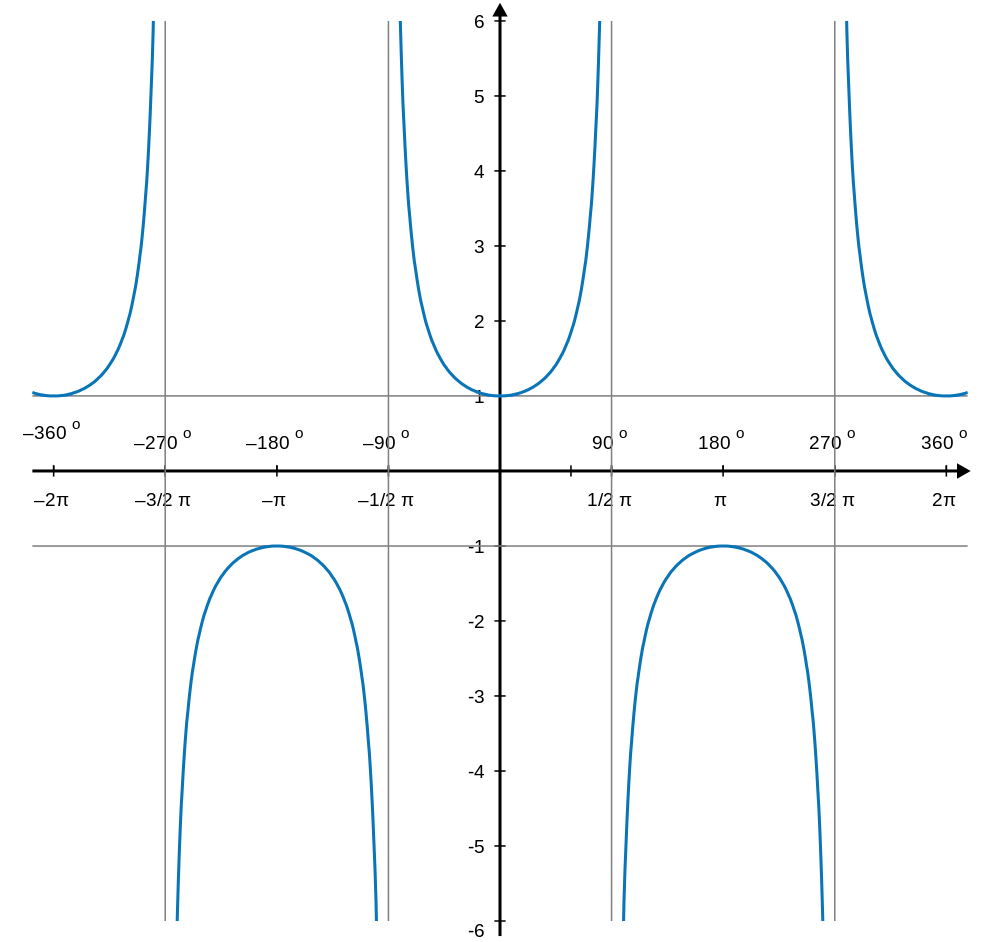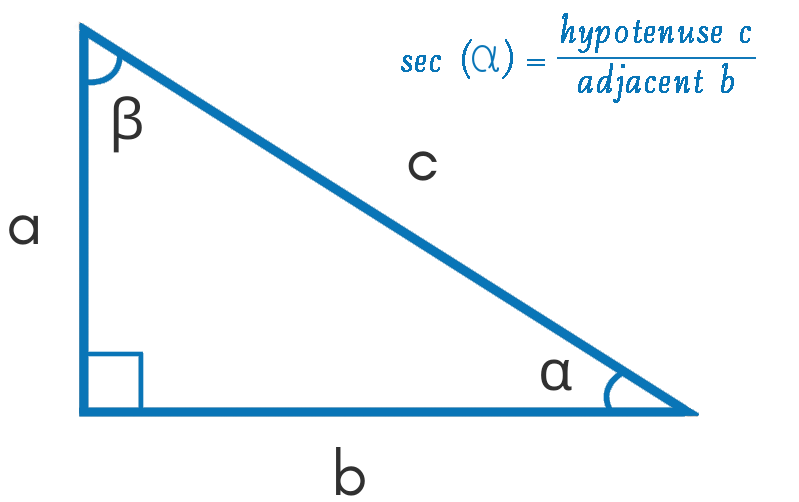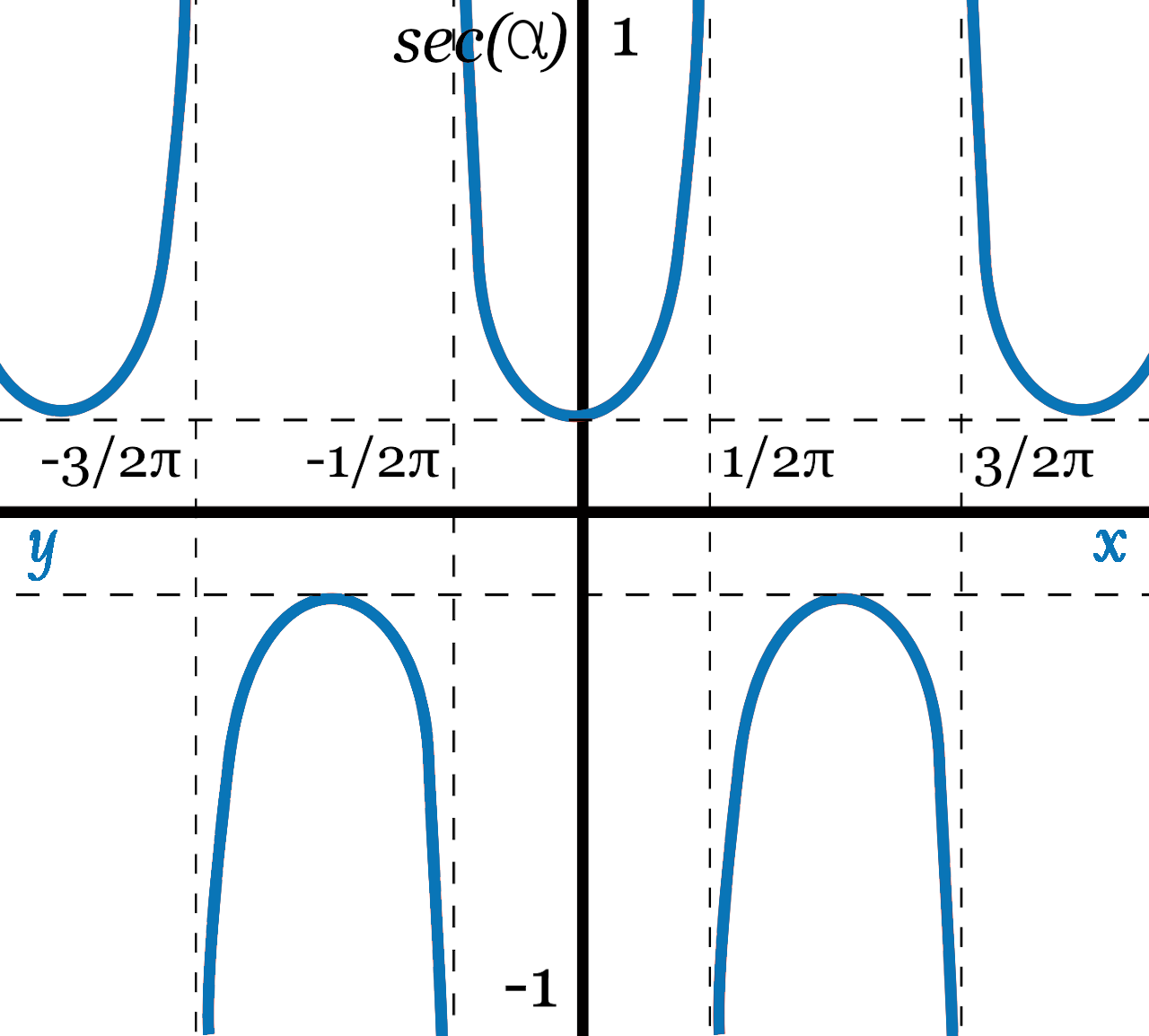Uh Oh! It seems you’re using an Ad blocker!

Since we’ve struggled a lot to makes online calculations for you, we are appealing to you to grant us by disabling the Ad blocker for this domain.# Secant Calculator

Angle (θ)Table of Content
 1 Secant Formula: 2 How To find Secant? 3 Table for Secant: 4 Graph of Secant: 5 How do you solve a Secant Equation? 6 What is Secant squared equal to? 7 Is SEC 2x the same as 1 Cos 2x? 8 What is the Secant Squared of 0?
Get The Widget!

Add Secant Calculator to your website to get the ease of using this calculator directly. Feel hassle-free to account this widget as it is 100% free, simple to use, and you can add it on multiple online platforms.

Available on App

The online secant calculator allows you to find the secant of the given angle in degree, radian, or the π radians. You can readily calculate the value of inverse secant (arcsec) trigonometric function by using this online free sec calculator.

Just give a couple of minutes to know what is secant equal to, how to find secant (sec), and much more that helps you in solving secant trig functions.

## What is Secant?

In trigonometry, there are a total of six ratios:

• Sine (sin θ)
• Cosine (cos θ)
• Tangent (tan θ)
• Secant (sec θ)
• Cosecant (cosec θ)
• Cotangent (cot θ)
From the above ratios, three are basic and three are the results of the division. Consequently, Secant is derived from the cosine ratio. In a right-angled triangle, the secant will be defined as the ratio of the length of the hypotenuse and the length of the adjacent side. To calculate secant, you will divide these two values. Additionally, it has a period of 2π as well. A sec calculator divided these two sides automatically to give you the value of secant.

Furthermore, an Online Arccos Calculator allows you to calculate the inverse of the cosine of an entered number.

### Secant Formula:

Formula for secant is:

$$sec(α) = hypotenuse c / adjacent b$$

You need to take a right triangle, then the secant of angle α will be equal to the length of the hypotenuse c that will be divided by the adjacent side b. However, a secant calculator is functioned to follow this formula automatically.

## How to Find Secant?

If you have a right triangle whose hypotenuse is 10 and adjacent is 2 then how will you calculate the secant of angle α?
For manual calculation, you have the formula and enter the given values in it. As the formula is:

• sec(α) = hypotenuse c / adjacent b.
• Given value of hypotenuse is = 10
• Given the value of adjacent side b = 2

So $$sec(α) = 10 / 2 = 5$$
However, a secant calculator won’t ask you to follow the above-mentioned steps. It will give you the required output just by filling the input fields. So, with the given picture you come to know what is secant equal to!## Table for Secant:

The table for secant displays all the common angles along with their secant values. To make quick calculations you can use secant tables and if the value of an angel is not present in the table then give a try to sec calculator to have the most accurate results.

 Angle (degrees) Angle (radians) Secant 0° 0 1 15° π/12 √6 – √2 30° π/6 2√3/3 45° π/4 √2 60° π/3 2 75° 5π/12 √6 + √2 90° π/2 undefined 105° 7π/12 -√6 – √2 120° 2π/3 -2 135° 3π/4 -√2 150° 5π/6 –2√3/3 165° 11π/12 -√6 – √2 180° Π -1 195° 13π/12 -√6 – √2 210° 7π/6 –2√3/3 225° 5π/4 -√2 240° 4π/3 -2 255° 17π/12 -√6 – √2 270° 3π/2 undefined 285° 19π/12 √6 + √2 300° 5π/3 2 315° 7π/4 √2 330° 11π/6 2√3/3 345° 23π/12 √6 – √2 360° 2π undefined

However, An Online Arcsin Calculator allows you to calculate the arcsin(x) and display the results in radians and degrees.

## Graph of Secant:

Whenever you will represent the secant function on the graph for every possible angle, there will come a series of repeating U-curves as shown below:Once the graph is plotted you will note that the secant of an angle will never be in the range of -1 to 1. Either it will be lesser than or equal to -1 or greater than or equal to 1. One other thing to focus on is that the curves won’t cross the x-axis.

## How Secant Calculator Works?

This sec calculator shows you how to find a secant quickly in two steps.

### Input:

• First of all, Enter the value of theta (angle α)
• Hit the Calculate Button

### Output:

The sec calculator will determine:

• The value of secant either in radians, degrees, or pi (π) radians, it depends on your selected angle units value

## FAQs:

### How do you solve a Secant Equation?

You can calculate secant by a secant calculator or by discovering the reciprocal of the cosine of an angle. The reciprocal of cos A will be 1 / cos A and the reciprocal of cos B will be 1 / cos B.  Consequently, sec A = 1/cos A and sec B= 1/cos B.

### What is Secant squared equal to?

The square of secant will be equals to:

• Square of tan function

We call it the secant squared formula as well as the square of secant function identity.

### Is SEC 2x the same as 1 Cos 2x?

Yes, it is already proven that these two terms are equal to each other. For the prove to observe the following equation:

• $$tan^-1 (√(a-x) /√(a+x)) = (cos^-1(x/a)) /2; -a<x<a$$

### What is the Secant Squared of 0?

If you take the secant square of zero it will be equal to 1. A square of the secant of 0 is 1² = 1.

## Final Note:

If your trigonometry test coming soon you can get a grip on such functions by utilizing this secant calculator. It will let you calculate the final output for any given value of the angel. Sec inverse calculator is free of cost for everyone and is well known for its quick and precise results. So let’s try to find the answer by putting the value of an angel in this calculator!

## References:

From the source of Wikipedia: Circles, Curves, Sets, and n-secants.

From the source of Oregon state: Two points determine a line, The slope of a secant line is a difference quotient.

From the source of Classaucsb: Secant Lines، Limit Definition of a Derivative، Average Rate of Change.